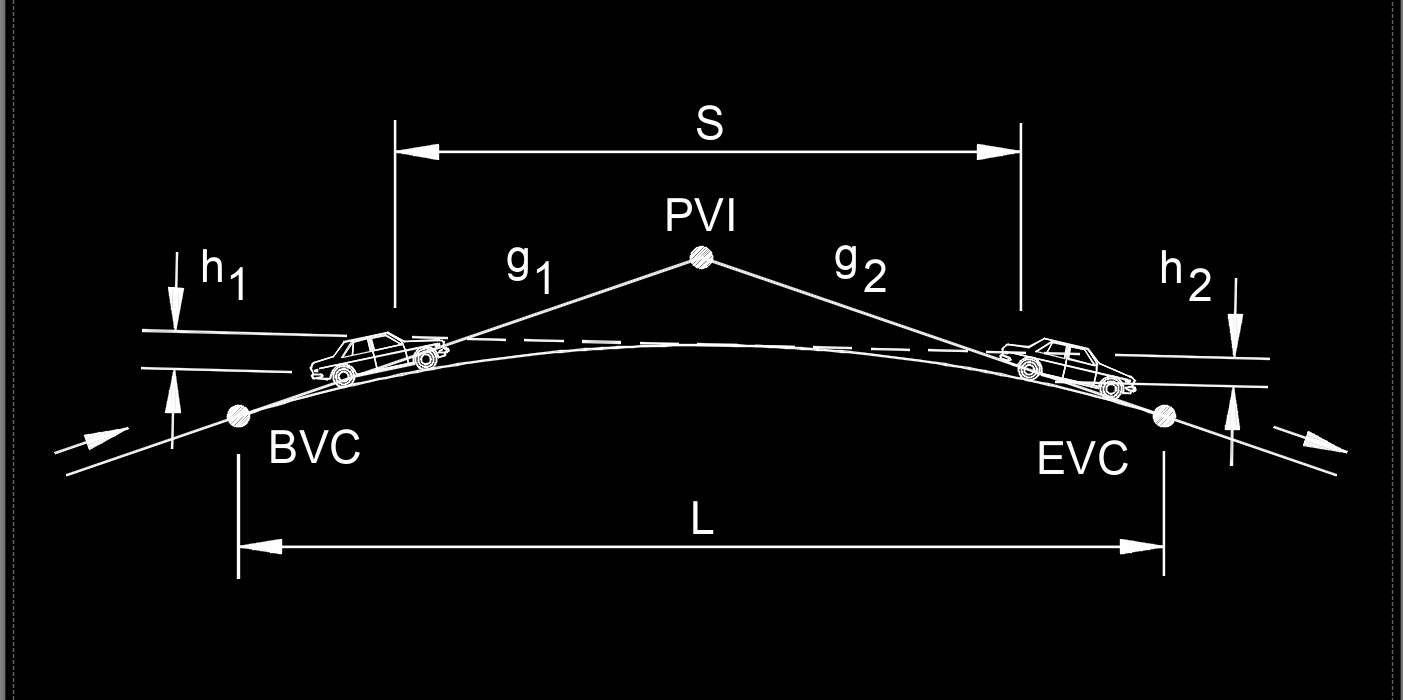# K Value

Written by Jerry Ratzlaff on . Posted in Civil EngineeringK value, abbreviated as K, is the horizontal distance required to achieve a 1% change in the slope of the vertical curve.

## K Value formula

 $$\large{ K = \frac{ L }{ A } }$$

### Where:

$$\large{ K }$$ = rate of vertical curvature

$$\large{ L }$$ = length of vertical curve

$$\large{ A }$$ = algebric difference in grade (%)